# Count Unique Values Using Pivot Table

This post will guide you how to count unique items in Excel Pivot Table. How do I use Pivot Table to count unique distinct values in Excel. How to get a distinct count in a pivot table in Excel.

Assuming that you have a list of 5 cells with 3 unique values, and you want to create an excel pivot table to count unique values, how to achieve it. You can insert a 3rd or helper column to check if the value is unique in the selected range of cells, and the create pivot table based on the 1st and 3rd column to count unique values. Or you can use the Distinct Count feature in pivot table to achieve the result, but this feature is only available in Excel 2013 and Excel 2016 version.

## Count Distinct Values Using Pivot Table with help column

#1 Insert 3rd column named as helper column (Column C), then type this formula into the Cell C2.

```=IF(SUMPRODUCT((\$A\$2:\$A2=A2)*(\$B\$2:\$B2=B2))>1,0,1)
```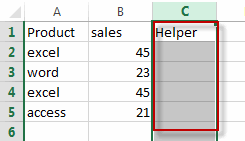#2 press Enter key in your keyboard, and drag the AutoFill Handler over other cells in helper column to apply this formula. If the returned value is 1, then it indicated that the value is the first value, if it returns 0, it indicated that the value is a duplicated value.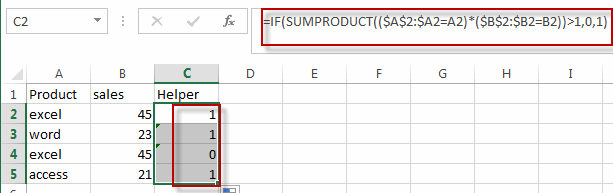#3 select the data to be included in a pivot table, the helper column should be also included.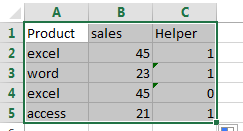#4 go to INSERT tab, click the PivotTable command under Tables group. The create PivotTable dialog will appear.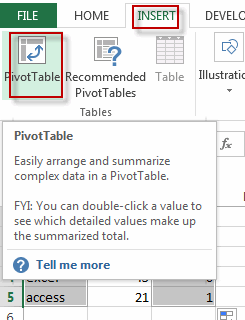#5 select Existing Worksheet radio button, and then select one cell or range to place the PivotTable report. Click OK button.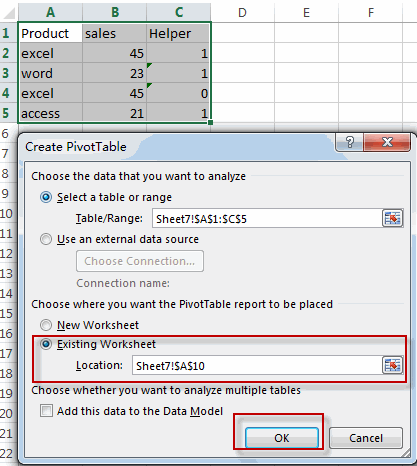#6 choose fields to add to report in PivotTable fields pane. Choose Product and Helper fields checkbox.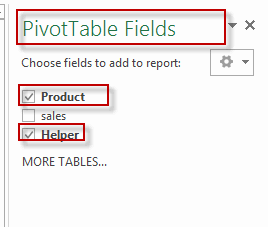#7 let’ see the result.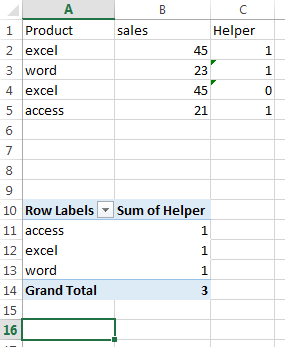## Count Distinct Values Using Pivot Table with distinct Count Feature

If you are using the latest version of Excel 2013 or Excel 2016, there is a new feature to allow you to count distinct values automatically in the pivot table. Let’s see the below detailed steps:

#1 select the range of cells that you want to be added into pivot table.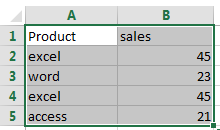#2 Click “INSERT” in the Ribbon tab, then clicking “Pivot Table” button in the “Tables” group. The Create PivotTable dialog will appear.#3 select Existing Worksheet radio button, and then select one cell or range to place the pivottable report. and select the Add this data to the Data Model checkbox. Click OK button.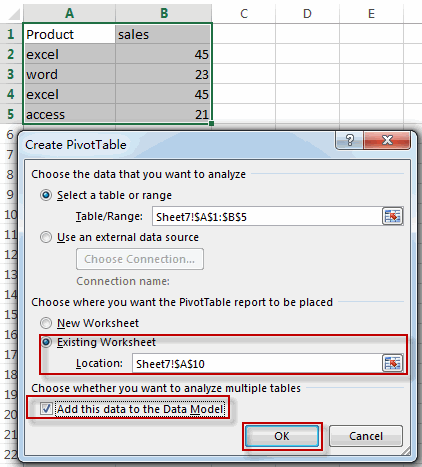#4 arrange the Rows, Columns and Values areas by dragging fields in PivotTable Fields pane.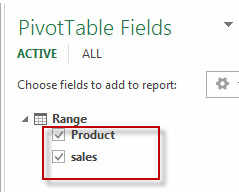#5 click on Values area, and select Value Field Settings from the drop down list. The Value Field Settings dialog will open.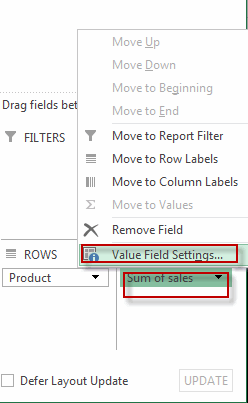#6 click Summarize Values By tab, and scroll down to Distinct Count, then click OK button.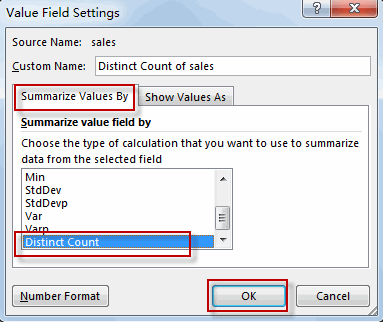#7 Let’s see the result.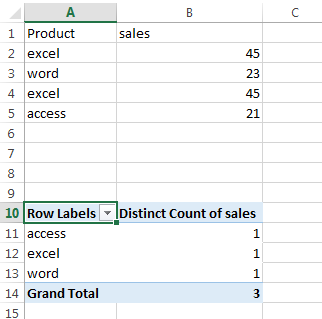### Related Functions

• Excel SUMPRODUCT function
The Excel SUMPRODUCT function multiplies corresponding components in the given one or more arrays or ranges, and returns the sum of those products.The syntax of the SUMPRODUCT function is as below:= SUMPRODUCT (array1,[array2],…)…
• Excel IF function
The Excel IF function perform a logical test to return one value if the condition is TRUE and return another value if the condition is FALSE. The IF function is a build-in function in Microsoft Excel and it is categorized as a Logical Function.The syntax of the IF function is as below:= IF (condition, [true_value], [false_value])….

Related Posts

Calculate Win Loss Tie

Suppose you got a task to calculate the win, loss, and tie totals; what would you do? If you are new to Ms Excel and don't have enough experience with it, then you might do this task manually but let ...

Count Cells that are Case Sensitive

If you are a frequent user of Microsoft Excel, you may have come across situations where you needed to add or count the cells that were case-sensitive. If you have done this task manually, I would like to point out ...

Check Dates in chronological order

Assume you have a date list that has different date formats, as seen in the accompanying picture. In this instance, Excel's Sort function will fail to sort them appropriately. However, you may convert all various date formats to a particular ...

Case Sensitive Lookup with SUMPRODUCT and EXACT

Today, we will show you how to use SUMPRODUCT and EXACT to perform a case sensitive exact match. In this article, we provide a simple example to calculate bonus for employees whose names are case-sensitive. If you meet similar scenarios ...

Average per Week by Formula in Excel

We usually apply AVERAGE function or relevant functions to return average directly in Excel worksheet. But in some situations, only applying average relevant functions cannot figure out our problem. Sometimes we can create a formula with functions and mathematical operation ...

How to Sum if Equal to Many Items or A Range in Excel

If we want to sum numbers from a range with criteria, we often select SUMIF of SUMIFS functions as the first choice to create a formula. The criteria can be a number or an array or a collection of some ...

How to Sum if Equal to X or Y in Excel

In daily work, if we want to sum numbers from a range, and only sum the numbers which being equal to X or Y in the range, we can create a formula with Excel build-in functions to get the result. ...

How To Sum the Largest N Values in Excel

Sometimes we may want to sum the largest N numbers or top N numbers in a range. In this article, we will show you the method of “SUM the Largest N Numbers” by a simple formula which consist of SUMPRODUCT ...

How to Sum for Cell Contains Formula Only in Excel

Sometimes values are created by formulas in cells. If we want to sum values which are created by formulas from a range, but some values which are hardcoded also list in the same range, how can we filter out matched ...

How to Sum the Smallest N Values in Excel

Sometimes we may want to sum the first smallest N numbers in a range in Excel. In this article, we will show you the method of “SUM the Smallest N Numbers” by a simple formula which consist of SUMPRODUCT and ...

Sidebar